# Connes-Consani for undergraduates (2)

Posted by on Oct 8, 2008 in Connes-Consani2008, undergraduateNo comments

Last time we have seen how an affine-algebra R gives us a maxi-functor (because the associated sets are typically huge)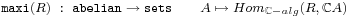Substantially smaller sets are produced from finitely generated-algebras S (therefore called mini-functors)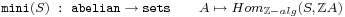Both these functors are ‘represented’ by existing geometrical objects, for a maxi-functor by the complex affine variety(the set of maximal ideals of the algebra R) with complex coordinate ring R and for a mini-functor by the integral affine scheme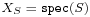(the set of all prime ideals of the algebra S).

The ‘philosophy’ of F_un mathematics is that an object over this virtual field with one elementrecords the essence of possibly complicated complex- or integral- objects in a small combinatorial thing.

For example, an n-dimensional complex vectorspace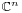has as its integral form a lattice of rank n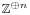. The corresponding-objects only records the dimension n, so it is a finite set consisting of n elements (think of them as the set of base-vectors of the vectorspace).

Similarly, all base-changes of the complex vectorspaceare given by invertible matrices with complex coefficients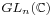. Of these base-changes, the only ones leaving the integral latticeintact are the matrices having all their entries integers and their determinant equal to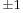, that is the group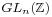. Of these integral matrices, the only ones that shuffle the base-vectors around are the permutation matrices, that is the group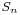of all possible ways to permute the n base-vectors. In fact, this example also illustrates Tits’ original motivation to introduce: the finite groupis the Weyl-group of the complex Lie group.

So, we expect a geometric-object to determine a much smaller functor from finite abelian groups to sets, and, therefore we call it a nano-functorbut as we do not know yet what the correct geometric object might be we will only assume for the moment that it is a subfunctor of some mini-functor. That is, for every finite abelian group A we have an inclusion of setsin such a way that these inclusions are compatible with morphisms. Again, take pen and paper and you are bound to discover the correct definition of what is called a natural transformation, that is, a ‘map’ between the two functors.

Right, now to make sense of our virtual F_un geometrical object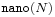we have to connect it to properly existing complex- and/or integral-geometrical objects.

Let us define a gadget to be a coupleconsisting of a nano- and a maxi-functor together with a ‘map’ (that is, a natural transformation) between them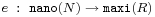The idea of this map is that it visualizes the elements of the set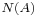as-points of the complex variety(that is, as a collection of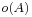points of, whereis the number of elements of).

In the example we used last time (the forgetful functor) with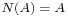any group-elementis mapped to the algebra mapin. On the geometry side, the points of the variety associated toare all algebra maps, that is, thecharacters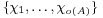. Therefore, a group-elementis mapped to the-point of the complex varietyconsisting of all character-values at: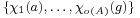.

In mathematics we do not merely consider objects (such as the gadgets defined just now), but also the morphisms between these objects. So, what might be a morphism between two gadgetsWell, naturally it should be a ‘map’ (that is, a natural transformation) between the nano-functorstogether with a morphism between the complex varieties(or equivalently, an algebra morphism) such that the extra gadget-structure (the evaluation maps) are preserved.

That is, for every finite Abelian groupwe should have a commuting diagram of mapsNot every gadget is a F_un variety though, for those should also have an integral form, that is, define a mini-functor. In fact, as we will see next time, an affine-variety is a gadget determining a unique mini-functor.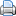Print This Post